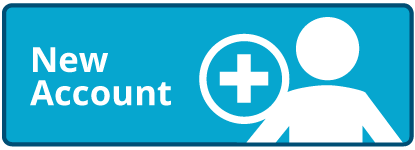Times up!
Times up! You may finish answering the current question and then proceed to your score!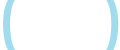Sorry, you have used all of your available hints for this lesson.
Practice Limit Reached

You've reached your daily practice limit of 12 questions.

Award InformationAwarded toQuestion HintChoose Family Member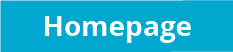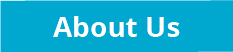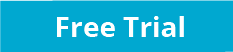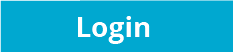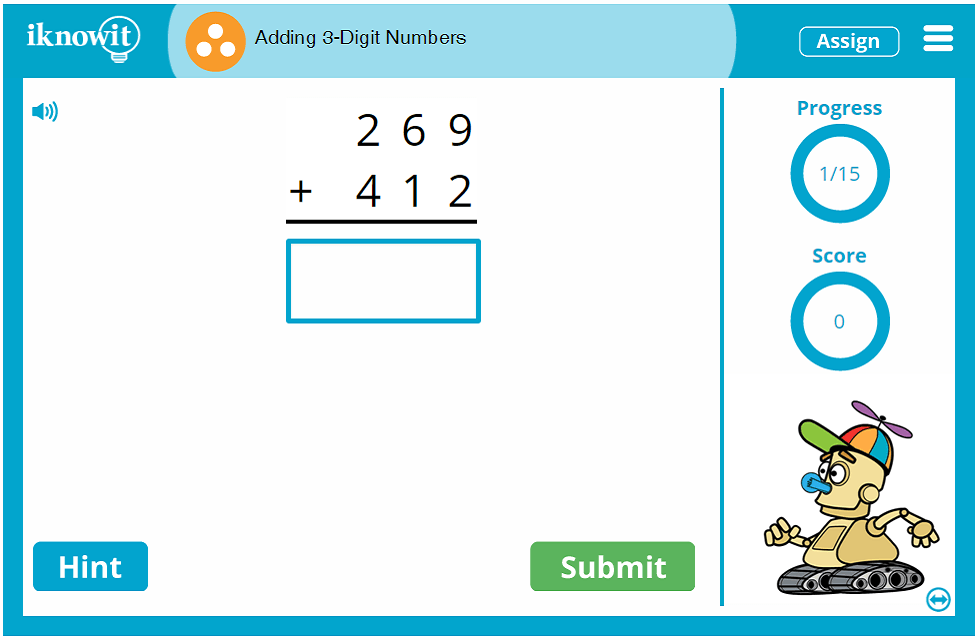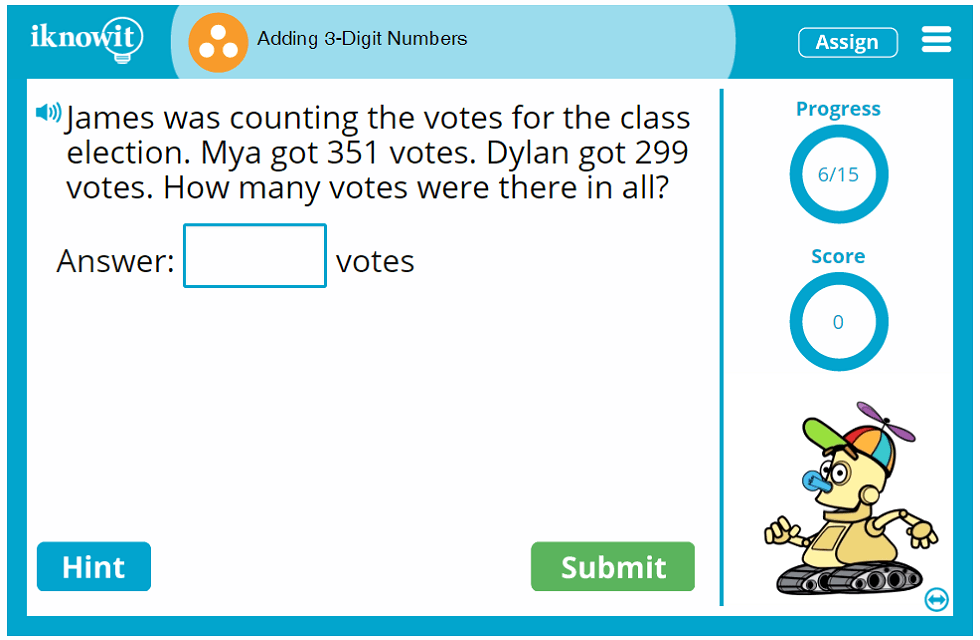## Online Math Lesson - Adding Three-Digit Numbers

It's time to practice adding three-digit numbers! Give this online math lesson from 'I Know It' a try! In this third grade-level math lesson, students will answer vertical addition problems, horizontal addition problems, and addition word problems involving three-digit numbers. Students will fill in the blank with the correct answer.

Addition with three-digit numbers can be a tricky skill to master, but this math lesson will help children soar to addition success! Soon, they will be pros at adding the ones, adding the tens, and adding the hundreds, including carrying numbers to the next place value!

Students might answer a word problem like this: "Xavier saved \$103. He earned \$407 babysitting. How much money does he have all together?" Or they might answer a horizontal addition problem like this: "347 + 139 =" You will find a variety of question types in this 3-digit addition lesson.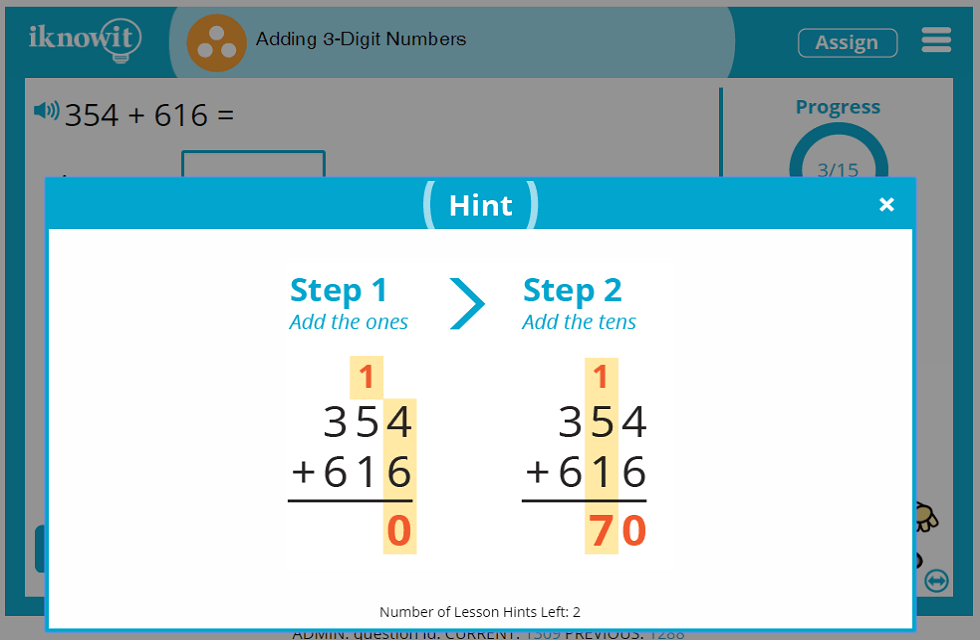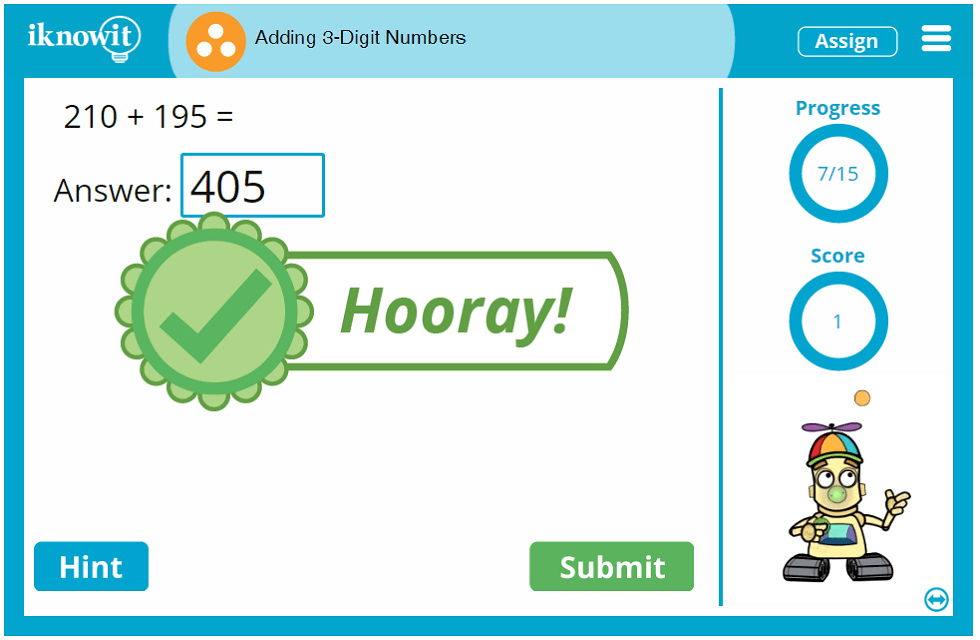'I Know It' is designed as an elementary-level math practice website. Our online math program is an ideal supplementary resource for your math curriculum. The kid-friendly graphics, easy-to-navigate layout, bright and exciting design, and animated characters make our program a delightful math practice experience for kids. Likewise, teachers appreciate the quality educational content reflected in each 'I Know It' math lesson.

'I Know It' online math games, including this adding 3-digit numbers lesson, include several features that help students make the most of their interactive math practice experience. The score-tracker on the right side of the screen tells students how many questions they've answered correctly so far. The progress-tracker above that tells them how many questions are left in the math lesson. The 'hint' button gives students a pictorial or written clue to help them solve the math problem. If they don't get the answer correct, a detailed explanation page pops up with step-by-step instructions on how to solve the question correctly, so they can apply what they've learned to the next problem.

You will notice that all math lessons on 'I Know It' have a speaker icon in the upper-left corner of the screen. This icon indicates the read-aloud feature; when pressed, the question will be read to the students in a clear voice. This feature is ideal for students who prefer auditory learning, or ESL/ELL students.

It is our sincere hope that this online math lesson about adding three-digit numbers will give your students extra confidence and proficiency in their basic addition skills. Be sure to try this online math game and browse our other math topics!

When you sign up for a free 30-day trial of 'I Know It', your students will be able to try this addition with three-digit numbers lesson at no cost! Students are able to answer a total of twenty-five questions per day across all math lessons on our website. For complete access to all the math lessons, administrative tools, and and features on our website, you will need to register as a member of iKnowIt.com.

There are so many benefits to being a member of iKnowIt.com! For example, you will be able to set up a class roster, add your students, and create individual user names and passwords for them to log in. You can also assign specific math lessons to individual students, check students' progress, print, email, and download progress reports, and change basic lesson settings (like limiting the number of hints per lesson and turning off the animations).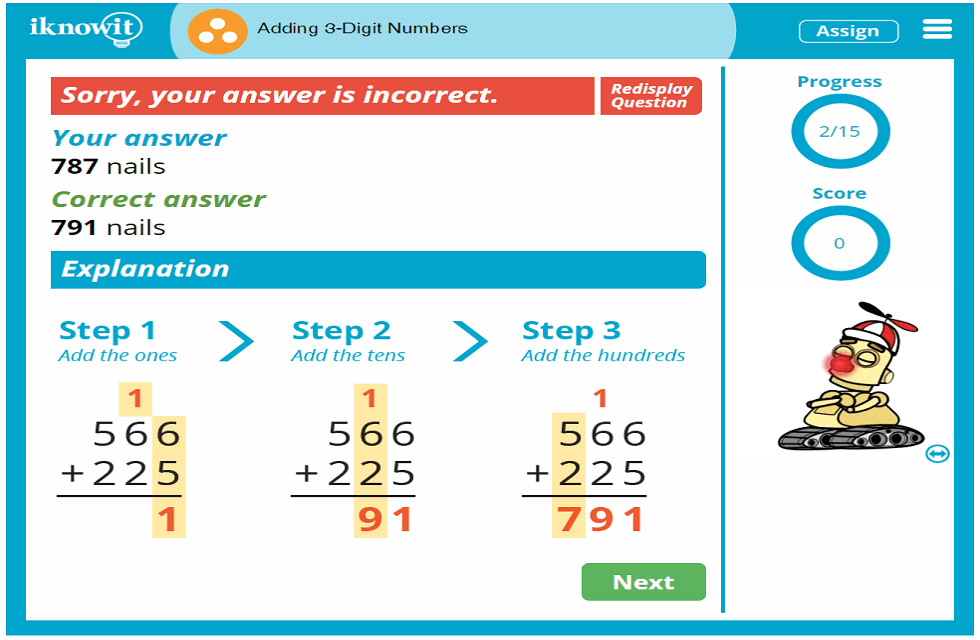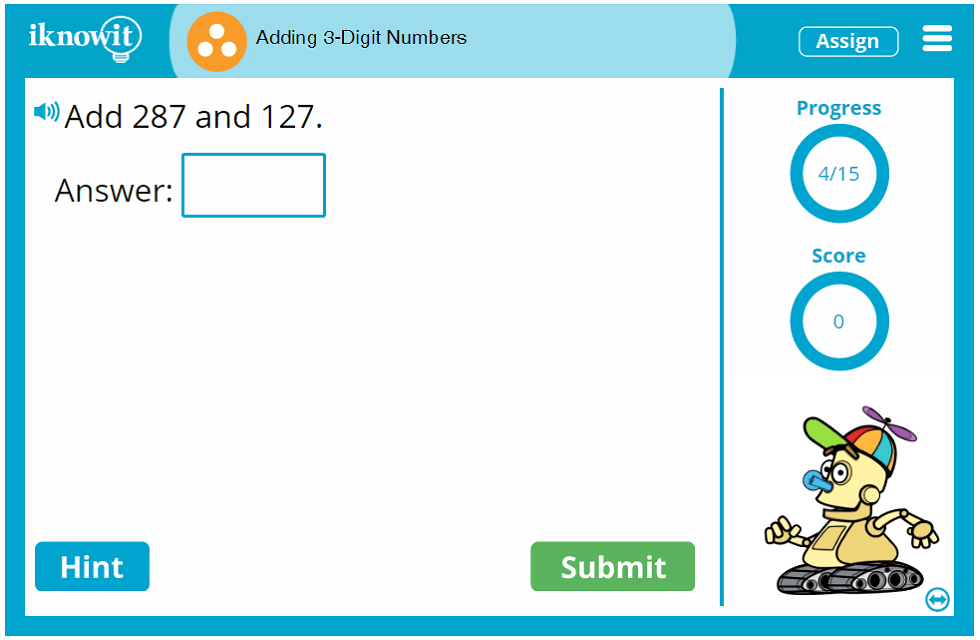## Level

This online math lesson featuring three-digit addition is categorized as a Level C lesson. You may want to try it out with your third grade or fourth grade class.

## Common Core Standard

3.NBT.2
Numbers and Operations in Base Ten

Students will put to use their understanding of place value and properties of operations to perform multi-digit arithmetic. This includes proficiency in adding or subtracting within one thousand based on their knowledge of place value, properties of operations operations, and the relationship between addition and subtraction.

## You might also be interested in...

In this third grade-level math lesson, students will practice adding four-digit numbers. They will solve problems in a vertical format, horizontal format, and addition word problems.

Adding 3-Digit and 4-Digit Numbers (Level D)
In this fourth grade-level math lessons, students will practice a mix of three-digit addition and four-digit addition. Problems will be shown in a vertical format, horizontal format, and word problem format.